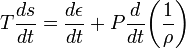User:Tohline/Math/EQ FirstLaw01$T \frac{ds}{dt} = \frac{d\epsilon}{dt} + P \frac{d}{dt} \biggl(\frac{1}{\rho}\biggr)$